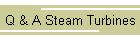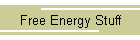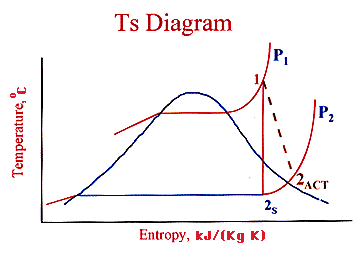## Isentropic Efficiency of a Steam Condensing TurbineThe Isentropic efficiency of a turbine is a comparison of the actual power output with the Isentropic case. Typical Isentropic efficiencies range from 70-90%.WhereTo calculate these enthalpy changes, you need to know the initial and final states, for example, temperature and pressure, of the working fluid for both the actual and isentropic cases. In the isentropic case, h2s is found from P2 and (S1 = S2s).

A typical Ts diagram for a steam turbine is shown below.REFERENCES : Jones, J. B., & R. E. Dugan. Engineering Thermodynamics.